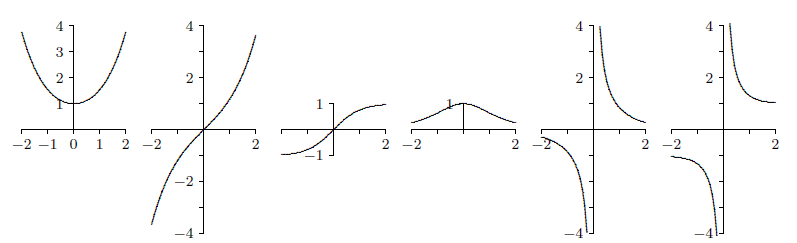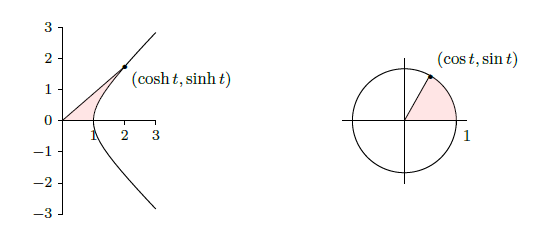$$\newcommand{\id}{\mathrm{id}}$$ $$\newcommand{\Span}{\mathrm{span}}$$ $$\newcommand{\kernel}{\mathrm{null}\,}$$ $$\newcommand{\range}{\mathrm{range}\,}$$ $$\newcommand{\RealPart}{\mathrm{Re}}$$ $$\newcommand{\ImaginaryPart}{\mathrm{Im}}$$ $$\newcommand{\Argument}{\mathrm{Arg}}$$ $$\newcommand{\norm}{\| #1 \|}$$ $$\newcommand{\inner}{\langle #1, #2 \rangle}$$ $$\newcommand{\Span}{\mathrm{span}}$$

# 4.11: Hyperbolic Functions

•• Contributed by David Guichard
• Professor (Mathematics) at Whitman College
$$\newcommand{\vecs}{\overset { \rightharpoonup} {\mathbf{#1}} }$$ $$\newcommand{\vecd}{\overset{-\!-\!\rightharpoonup}{\vphantom{a}\smash {#1}}}$$$$\newcommand{\id}{\mathrm{id}}$$ $$\newcommand{\Span}{\mathrm{span}}$$ $$\newcommand{\kernel}{\mathrm{null}\,}$$ $$\newcommand{\range}{\mathrm{range}\,}$$ $$\newcommand{\RealPart}{\mathrm{Re}}$$ $$\newcommand{\ImaginaryPart}{\mathrm{Im}}$$ $$\newcommand{\Argument}{\mathrm{Arg}}$$ $$\newcommand{\norm}{\| #1 \|}$$ $$\newcommand{\inner}{\langle #1, #2 \rangle}$$ $$\newcommand{\Span}{\mathrm{span}}$$ $$\newcommand{\id}{\mathrm{id}}$$ $$\newcommand{\Span}{\mathrm{span}}$$ $$\newcommand{\kernel}{\mathrm{null}\,}$$ $$\newcommand{\range}{\mathrm{range}\,}$$ $$\newcommand{\RealPart}{\mathrm{Re}}$$ $$\newcommand{\ImaginaryPart}{\mathrm{Im}}$$ $$\newcommand{\Argument}{\mathrm{Arg}}$$ $$\newcommand{\norm}{\| #1 \|}$$ $$\newcommand{\inner}{\langle #1, #2 \rangle}$$ $$\newcommand{\Span}{\mathrm{span}}$$

The hyperbolic functions appear with some frequency in applications, and are quite similar in many respects to the trigonometric functions. This is a bit surprising given our initial definitions.

Definition 4.11.1: Hyperbolic Cosines and Sines

The hyperbolic cosine is the function

$\cosh x ={e^x +e^{-x }\over2},$

and the hyperbolic sine is the function

$\sinh x ={e^x -e^{-x}\over 2}.$

Notice that $$\cosh$$ is even (that is, $$\cosh(-x)=\cosh(x)$$) while $$\sinh$$ is odd ($$\sinh(-x)=-\sinh(x)$$), and $$\cosh x + \sinh x = e^x$$. Also, for all $$x$$, $$\cosh x >0$$, while $$\sinh x=0$$ if and only if $$e^x -e^{-x }=0$$, which is true precisely when $$x=0$$.

Lemma 4.11.2

The range of $$\cosh x$$ is $$[1,\infty)$$.

Proof

Let $$y= \cosh x$$. We solve for $$x$$:

\eqalign{y&={e^x +e^{-x }\over 2}\cr 2y &= e^x + e^{-x }\cr 2ye^x &= e^{2x} + 1\cr 0 &= e^{2x}-2ye^x +1\cr e^{x} &= {2y \pm \sqrt{4y^2 -4}\over 2}\cr e^{x} &= y\pm \sqrt{y^2 -1}\cr}

From the last equation, we see $$y^2 \geq 1$$, and since $$y\geq 0$$, it follows that $$y\geq 1$$.

Now suppose $$y\geq 1$$, so $$y\pm \sqrt{y^2 -1}>0$$. Then $$x = \ln(y\pm \sqrt{y^2 -1})$$ is a real number, and $$y =\cosh x$$, so $$y$$ is in the range of $$\cosh(x)$$.

$$\square$$

Definition 4.11.3: Hyperbolic Tangent and Cotangent

The other hyperbolic functions are

\eqalign{\tanh x &= {\sinh x\over\cosh x}\cr \coth x &= {\cosh x\over\sinh x}\cr \text{sech} x &= {1\over\cosh x}\cr \text{csch} x &= {1\over\sinh x}\cr}

The domain of $$\coth$$ and $$\text{csch}$$ is $$x\neq 0$$ while the domain of the other hyperbolic functions is all real numbers. Graphs are shown in Figure $$\PageIndex{1}$$Figure $$\PageIndex{1}$$: The hyperbolic functions.

Certainly the hyperbolic functions do not closely resemble the trigonometric functions graphically. But they do have analogous properties, beginning with the following identity.

Theorem 4.11.4

For all $$x$$ in $$\mathbb{R}$$, $$\cosh ^2 x -\sinh ^2 x = 1$$.

Proof

The proof is a straightforward computation:

$\cosh ^2 x -\sinh ^2 x = {(e^x +e^{-x} )^2\over 4} -{(e^x -e^{-x} )^2\over 4}= {e^{2x} + 2 + e^{-2x } - e^{2x } + 2 - e^{-2x}\over 4}= {4\over 4} = 1.$

$$\square$$

This immediately gives two additional identities:

$1-\tanh^2 x =\text{sech}^2 x\qquad\hbox{and}\qquad \coth^2 x - 1 =\text{csch}^2 x.$

The identity of the theorem also helps to provide a geometric motivation. Recall that the graph of $$x^2 -y^2 =1$$ is a hyperbola with asymptotes $$x=\pm y$$ whose $$x$$-intercepts are $$\pm 1$$. If $$(x,y)$$ is a point on the right half of the hyperbola, and if we let $$x=\cosh t$$, then $$y=\pm\sqrt{x^2-1}=\pm\sqrt{\cosh^2x-1}=\pm\sinh t$$. So for some suitable $$t$$, $$\cosh t$$ and $$\sinh t$$ are the coordinates of a typical point on the hyperbola. In fact, it turns out that $$t$$ is twice the area shown in the first graph of Figure $$\PageIndex{2}$$. Even this is analogous to trigonometry; $$\cos t$$ and $$\sin t$$ are the coordinates of a typical point on the unit circle, and $$t$$ is twice the area shown in the second graph of Figure $$\PageIndex{2}$$.Figure $$\PageIndex{2}$$: Geometric definitions of sin, cos, sinh, cosh: $$t$$ is twice the shaded area in each figure.

Given the definitions of the hyperbolic functions, finding their derivatives is straightforward. Here again we see similarities to the trigonometric functions.

Theorem 4.11.5

$${d\over dx}\cosh x=\sinh x$$ and \thmrdef{thm:hyperbolic derivatives} $${d\over dx}\sinh x = \cosh x$$.

Proof

${d\over dx}\cosh x= {d\over dx}{e^x +e^{-x}\over 2} = {e^x- e^{-x}\over 2} =\sinh x,$

and

${d\over dx}\sinh x = {d\over dx}{e^x -e^{-x}\over 2} = {e^x +e^{-x }\over 2} =\cosh x.$

$$\square$$

Since $$\cosh x > 0$$, $$\sinh x$$ is increasing and hence injective, so $$\sinh x$$ has an inverse, $$\text{arcsinh} x$$. Also, $$\sinh x > 0$$ when $$x>0$$, so $$\cosh x$$ is injective on $$[0,\infty)$$ and has a (partial) inverse, $$\text{arccosh} x$$. The other hyperbolic functions have inverses as well, though $$\text{arcsech} x$$ is only a partial inverse. We may compute the derivatives of these functions as we have other inverse functions.

Theorem 4.11.6

$${d\over dx}\text{arcsinh} x = {1\over\sqrt{1+x^2}}$$.

Proof

Let $$y=\text{arcsinh} x$$, so $$\sinh y=x$$. Then

${d\over dx}\sinh y = \cosh(y)\cdot y' = 1,$

and so

$y' ={1\over\cosh y} ={1\over\sqrt{1 +\sinh^2 y}} = {1\over\sqrt{1+x^2}}.$

$$\square$$

The other derivatives are left to the exercises.

## Contributors

• Integrated by Justin Marshall.